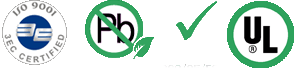# Maximal current load of wire

Allowable current load of the wire as a function of temperature riseExample of the calculation : Required current is 8 Amps with maximal 30°C temperature rise of. How wide wire shall I use on board with 35µm Cu foil? In graph we find point A, which refers to 8 Amps current and intersect with 30°C temperature rise curve (point B ). Then we connect point B with bottom curve, which refers to Cu foil of 35µm (point C ). From this point C we can read required width of the wire (point D ). In this case it is 2.5 mm More information can be found here : www.ultracad.com

Wire width equation (Rovnice výpočtu šíře vodiče) :

I = 0.0150(DT 0.5453)(A 0.7349) for norm IPC-D-275 (inner layers wires)

I = 0.0647(DT 0.4281)(A 0.6732) for norm IPC-D-275 (outer layers wires)

where:

I = maximal current in Amps

DT = temperature rise in °C

A = Area of cross section of the wire in square milscompany adress :
Žateckých 1532/7
140 00 Praha 4, Česká republika
...map...place of business :
Technologický park Běchovice
190 11 Praha 9, Česká republika
...map...

tel: + 420 222 729 257, + 420 222 590 402
fax: + 420 222 724 032
email:internet: